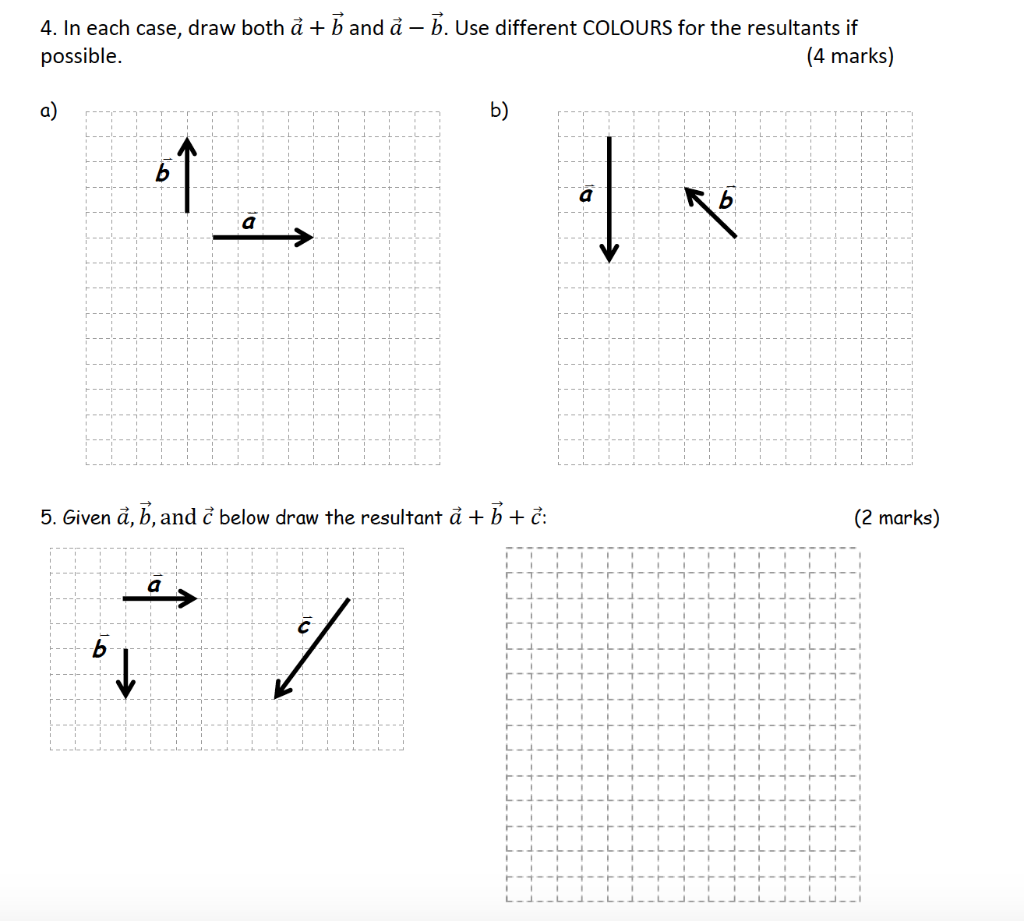# Question Solved1 Answer4. In each case, draw both ả + ħ and à – Đ. Use different COLOURS for the resultants if possible. (4 marks) a) b) ko a 5. Given å, b, and ĉ below draw the resultant à ++ : (2 marks) a ins /H8P7M5 The Asker · Mechanical EngineeringTranscribed Image Text: 4. In each case, draw both ả + ħ and à – Đ. Use different COLOURS for the resultants if possible. (4 marks) a) b) ko a 5. Given å, b, and ĉ below draw the resultant à ++ : (2 marks) a ins /
More
Transcribed Image Text: 4. In each case, draw both ả + ħ and à – Đ. Use different COLOURS for the resultants if possible. (4 marks) a) b) ko a 5. Given å, b, and ĉ below draw the resultant à ++ : (2 marks) a ins /